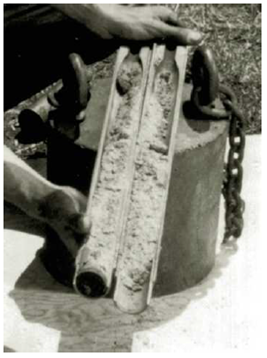Chapter 3, Problem 3.12PPrinciples of Geotechnical Enginee...

9th Edition
Braja M. Das + 1 other
ISBN: 9781305970939

Solutions

Chapter
SectionPrinciples of Geotechnical Enginee...

9th Edition
Braja M. Das + 1 other
ISBN: 9781305970939
Textbook Problem

Refer to Problem 3.11. A 3-in. long specimen was cut from the split-spoon sampler for performing a shear strength test. If the specimen is required to be 100% saturated for the test, determine:a. Saturated unit weightb. Moisture content at 100% saturationc. Amount of water (in lb) needed to achieve full saturation3.11 During a subsurface exploration, an undisturbed soil sample was collected from the field using a split-spoon sampler for laboratory evaluation (see Figure 17.6 in Chapter 17). The sample has a diameter of 1.375 in., length of 18 in., and a moist weight of 1.85 lb. If the oven-dried weight was 1.5 lb and Gs = 2.74, calculate the following:a. Moist unit weightb. Moisture contentc. Dry unit weightd. Void ratioe. Degree of saturationFigure 17.6 Split-spoon sampler, unassembled (Courtesy of ELE International)

(a)

To determine

Calculate the saturated unit weight of soil.

Explanation

Given information:

The diameter of the sample (d) is 1.375in..

The length of the sampler (L) is 18in..

The length of the sampler for performing shear strength (Lshear) is 3in..

The moist weight (W) of the sample is 1.85lb.

The oven-dried weight (WS) of the sample is 1.5lb.

The specific gravity of the soil solids (Gs) is 2.74.

Calculation:

Consider the unit weight of water (γw) is 62.4lb/ft3.

Calculate the volume of the sampler (V) using the relation.

V=14πd2L

Substitute 1.375in. for d and 18in. for L.

V=14π×1.3752×18=26.728in.3×(1ft12in.)3=0.015ft3

Calculate the moist unit weight of the soil (γ) using the relation.

γ=WV

Substitute 1.85lb for W and 0.015ft3 for V.

γ=1.850.015=123.33lb/ft3

Calculate the moisture content (w) using the relation.

w=WWsWs

Substitute 1.85lb for W and 1.5lb for Ws .

w=1.851.51.5=0.351.5=0.2333×100%=23

(b)

To determine

Calculate the moisture content at 100% saturation.

(c)

To determine

Calculate the amount of water needed to achieve full saturation.

Still sussing out bartleby?

Check out a sample textbook solution.

See a sample solution

The Solution to Your Study Problems

Bartleby provides explanations to thousands of textbook problems written by our experts, many with advanced degrees!

Get Started

Why is important to know integral calculus?

Engineering Fundamentals: An Introduction to Engineering (MindTap Course List)

What is a table, and what role does it play in the relational model?

Database Systems: Design, Implementation, & Management

What can be done on the job site to reduce the danger of reflected light?

Welding: Principles and Applications (MindTap Course List)